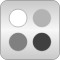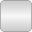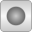# CompressibleLiquids

Compressible liquid models# Package ContentsCommon Base classes for compressible liquidsLinearColdWater Cold water model with linear compressibilityLinearWater_pT_Ambient Liquid, linear compressibility water model at 1.01325 bar and 25 degree Celsius

# Information

This information is part of the Modelica Standard Library maintained by the Modelica Association.

#### Fluid models with linear compressibility, using PartialLinearFluid as base class.

The linear compressibility fluid models contained in this package are based on the assumptions that:

• The specific heat capacity at constant pressure (cp) is constant
• The isobaric expansion coefficient (beta) is constant
• The isothermal compressibility (kappa) is constant
• Pressure and temperature are used as states

This results in models that are only valid for small temperature ranges, but sufficient to model compressibility and e.g., the "water hammer" effect. Another advantage id that only 3 values need to be measured to have an initial model. Hydraulic fluids can often be approximated by this type of model.

That means that the density is a linear function in temperature and in pressure. In order to define the complete model, a number of constant reference values are needed which are computed at the reference values of the states pressure p and temperature T. The model can be interpreted as a linearization of a full non-linear fluid model (but it is not linear in all thermodynamic coordinates). Reference values are needed for

1. the density (reference_d),
2. the specific enthalpy (reference_h),
3. the specific entropy (reference_s).

Apart from that, a user needs to define the molar mass, MM_const. Note that it is possible to define a fluid by computing the reference values from a full non-linear fluid model by computing the package constants using the standard functions defined in a fluid package (see example in Common, LinearWater_pT).